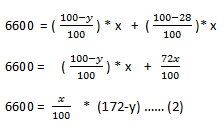New Students Offer - Use Code HELLO

# Data Interpretation For IBPS PO 2017: Part 2

Direction (1-3)There are three shops A,B and C which sells different articles namely I , II III , IV and V respectively.the discount offered by each shops on different articles is given in the table.

 ARTICLES DISCOUNT % BY SHOP A DISCOUNT % BY SHOP B DISCOUNT % BY SHOP C I 26% 28% 34% II 14% -- 28% III -- 12% -- IV 26% 34% -- V -- -- 24%

1) If the average selling price of an article I on all shops is Rs. 4293 , then find the selling price of article I in shop B
a)4912
b)4523
c)4374
d)3416
e)4312

2) If the average selling price of article II in shop A and B is Rs. 3608 and that in shop B and C is Rs. 3300 , then the discount offered by shop B( in rupees) is ?
a) 968
b)732
c) 1734
d)862
e)1324

3) If a new shop D come now and started selling article III with some percent discount whose amount is equal to the amount of discount offered by shop C in article II (given that MP decided by shop D is 66 2/ 3 % more than that by shop c ).Find the percentage of discount offered by shop D.
a)18.66 %
b) 18.33%
c)15%
d) 17%
e)23%

#### 1) c

Clearly given MP (market price ) will be same for all shops , so lets take market price to be “ x”
Now SP = MP * ( 100 – discount ) / 100 (Or) SP= x * (100 – discount) / 100
(we all know we sell a product after deducting its discount percentage from the total percentage )
In the given question average selling price is given i.e. 4293 for 3 shops , so the total price of all the three after the discount will be 4293 * 3 = 12879
Writing in equation form ,(SP of A = (100 – discount ) * x / 100 = (100 -26)* x / 100 = 74x/ 100 Similiarly for B and C )x = 12879 * 100 / 212
marked price (x)= 6075
we found the marked price , next step is to find the selling price of ARTICLE I by shop BSP= 72 * 6075 / 100
Sp = 4374

#### 2) a

average selling price of article II by shop A and B is 3608 , so total selling price of both will be 7216
Writing in equation form :
Let “x “ be the market price which is same for all shops , discount rate of A for article II is 14 % , let “y “ be the discount rate for shop BAlso ,
average selling price of article II by shop B and C is 3300 , so total selling price of both will be 6600
Writing in equation form :
Let “x “ be the market price which is same for all shops , discount rate of C for article II is 28 % , let “y “ be the discount rate for shop B.Subtracting both equations , we getSo market price = 4400
Discount offer by B can be found by substituting x in any one of the equation
Y = 22%
Disount amount = 4400 * 22 / 100
Discount amount = 968 , so (a) is the correct choice

#### 3) c

MP II is the market price of article II and (MPD ) is the market price of D
given ,26% of MP II = x % (MPD ) ….. (1)
suppose if market price of article II by shop c is 100 % then , for shop D will be
(MPD ) is 100 + 66 2/3% = 500/3% of MPC
(MPD ) / MPC = 500/300 = 5/3
So substituting this in the equation 1
We get 100 cancelled ,
26 MP II = x(MPD )
(MPD ) 26*3/5 = x
X= 15.35
So (c) 15 is the nearest in the answer and the correct choice.